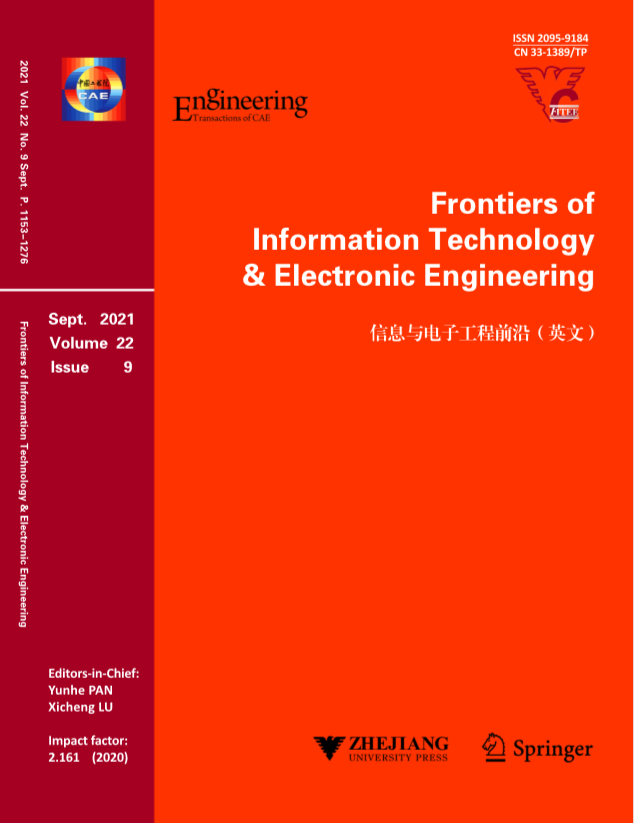,     et al.

,     et al.

,     et al.

,     et al.

,     et al.

,     et al.

,     et al.

,     et al.

,     et al.

,     et al.

,     et al.

,     et al.

,     et al.

,     et al.

,     et al.

,     et al.

,     et al.

,     et al.

,     et al.

,     et al.

,     et al.

,     et al.

#### Effective and selective separation of perrhenate from acidic wastewater by super-stable, superhydrophobic, and recyclable biosorbent#### Frontiers of Information Technology & Electronic Engineering

Volume 22,  Issue 9

,     et al.

,     et al.

,     et al.

,     et al.

,     et al.

,     et al.

,     et al.

,     et al.

,     et al.

,     et al.

,     et al.

,     et al.

,     et al.